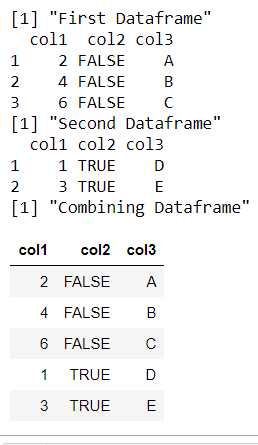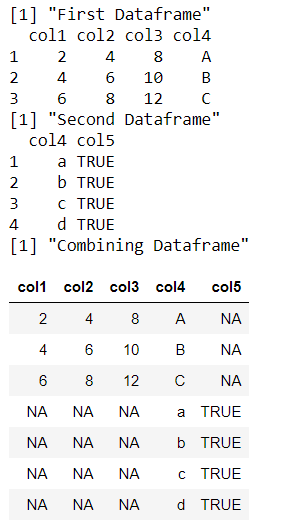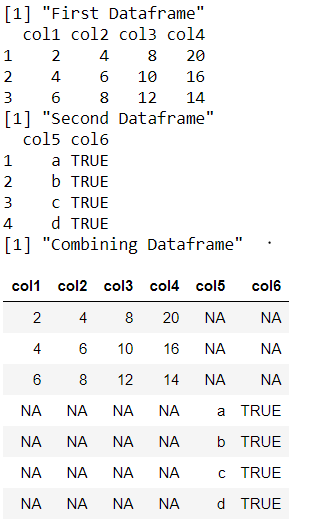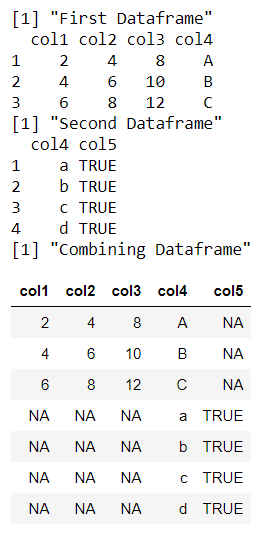Related Articles

# How to add dataframe to dataframe in R ?

• Last Updated : 13 Jul, 2021

In this article, we will see how to add dataframe at the end of another dataframe in R Programming Language.

## Method 1: Using rbind() method

The rbind() method in R works only if both the input dataframe contains the same columns with similar lengths and names. The dataframes may have a different number of rows. The class of the dataframe columns should be consistent with each other, otherwise, errors are thrown. The following properties are maintained :

• The rows are appended in the order of dataframe appearance during the function call.
• The total number of columns is equivalent to the columns in any of the dataframes.
• The total number of rows is equivalent to the summation of rows in the dataframe.
`rbind ( df1, df2)`

Example: Adding Dataframe using rbind() method

## R

 `# declaring first dataframe``data_frame1 <- ``data.frame``(col1 = ``c``(2, 4, 6),``                          ``col2 = ``FALSE` `,``                          ``col3 = ``LETTERS``[1 : 3])`` ` `print ``(``"First Dataframe"``)``print ``(data_frame1)`` ` `# declaring second dataframe``data_frame2 <- ``data.frame``(col1 = ``c``(1 , 3),``                          ``col2 = ``TRUE``, ``                          ``col3 = ``LETTERS``[4 : 5])`` ` `print ``(``"Second Dataframe"``)``print ``(data_frame2)``print ``(``"Combining Dataframe"``)`` ` `# binding dataframes``rbind``(data_frame1, data_frame2)`

Output:## Method 2: Using plyr package

The “plyr” package in R is used to work with data, including its enhancements and manipulations. It can be loaded and installed into the working space by the following command:

`install.packages("plyr")`

rbind.fill() method in R is an enhancement of the rbind() method in base R, is used to combine dataframes with different columns. The column names are numbers may be different in the input dataframes. Missing columns of the corresponding dataframes are filled with NA. The output dataframe contains a column only if it is present in any of the dataframes.

`rbind.fill( df1, df2)`

The following properties are maintained by the rbind.fill() method :

• The dataframes are appended in the order of their specification in the function.
• The total number of columns is equivalent to the summation of the number of columns of both the dataframes.
• The total number of rows is equivalent to the summation of the number of rows of both the dataframes.
• The appearance of columns is in the order of dataframe arguments declaration during the function call.
• Empty cells are created in the missing columns.

Example: Adding Dataframe using plyr package

## R

 `# loading the required library``library``(``"plyr"``)`` ` `# declaring first dataframe``data_frame1 <- ``data.frame``(col1 = ``c``(2, 4, 6),``                          ``col2 = ``c``(4, 6, 8),``                          ``col3 = ``c``(8, 10, 12),``                          ``col4 = ``LETTERS``[1 : 3])`` ` `print ``(``"First Dataframe"``)``print ``(data_frame1)`` ` `# declaring second dataframe``data_frame2 <- ``data.frame``(col4 = ``letters``[1:4],``                          ``col5 = ``TRUE``)`` ` `print ``(``"Second Dataframe"``)``print ``(data_frame2)``print ``(``"Combining Dataframe"``)`` ` `# binding dataframes``rbind.fill``(data_frame1, data_frame2)`

Output:## Method 3: Using dplyr package

The “dplyr” package in R is used to work with data, including its enhancements and manipulations. It can be loaded and installed into the working space by the following command :

`install.packages("dplyr")`

The bind_rows() method is used to combine dataframes with different columns. The column names are numbers may be different in the input dataframes. Missing columns of the corresponding dataframes are filled with NA. The output dataframe contains a column only if it is present in any of the dataframes.

`bind_rows(df1, df2)`

The properties are similar to the working of the “plyr” package. This method just offers an enhancement to data manipulation.

Example: Adding Dataframe using dplyr package

## R

 `# loading the required library``library``(``"dplyr"``)`` ` `# declaring first dataframe``data_frame1 <- ``data.frame``(col1 = ``c``(2, 4, 6),``                          ``col2 = ``c``(4, 6, 8),``                          ``col3 = ``c``(8, 10, 12),``                          ``col4 = ``c``(20, 16, 14))`` ` `print ``(``"First Dataframe"``)``print ``(data_frame1)`` ` `# declaring second dataframe``data_frame2 <- ``data.frame``(col5 = ``letters``[1 : 4],``                          ``col6 = ``TRUE``)`` ` `print ``(``"Second Dataframe"``)``print ``(data_frame2)``print ``(``"Combining Dataframe"``)`` ` `# binding dataframes``bind_rows``(data_frame1, data_frame2)`

Output:In case, any of the column names are the same in both of the input dataframes, then the following properties are encountered :

• The class of the common column should be the same in both the dataframes, otherwise, an error is encountered.

In this case, the total number of columns in the output dataframe should be equivalent to the total input columns minus the intersecting columns.

Example: Adding dataframe with one column having same name.

## R

 `# loading the required library``library``(``"dplyr"``)`` ` `# declaring first dataframe``data_frame1 <- ``data.frame``(col1 = ``c``(2, 4, 6),``                          ``col2 = ``c``(4, 6, 8),``                          ``col3 = ``c``(8, 10, 12),``                          ``col4 = ``LETTERS``[1 : 3])`` ` `print ``(``"First Dataframe"``)``print ``(data_frame1)`` ` `# declaring second dataframe``data_frame2 <- ``data.frame``(col4 = ``letters``[1 : 4],``                          ``col5 = ``TRUE``)``print ``(``"Second Dataframe"``)``print ``(data_frame2)``print ``(``"Combining Dataframe"``)`` ` `# binding dataframes``bind_rows``(data_frame1,data_frame2)`

Output:My Personal Notes arrow_drop_up